• 它还包含一些协方差函数： - 1: 母 3/2 - 2: 母 5/2 - 3：神经网络- 4：定期- 5：平方指数。 - 6: Matern + 平方指数- 7: Matern 3/2 + 神经网络高斯过程回归先验知识是 U、观测值 F、协方差（数字）COV、评估变量 ...matlab
• 用c语言实现协方差函数，方便c语言算法的学习。把c语言用在应用数学上，解析数学公式。
• 一种新的求最小二乘配置协方差函数及其在GPS高程拟合中的应用，李军海，文汉江，在用最小二乘配置对高程异常拟合过程中关键是预先知道各信号间的协方差，这里提出一种新的求协方差函数模型的方法，即球状函数模
• 半变异函数和协方差函数将邻近事物比远处事物更相似这一假设加以量化。半变异函数和协方差都将统计相关性的强度作为距离函数来测量。
• C 协方差 数值代数 原创 开发技术 代码 函数可以直接调用的
• 文章目录期望方差协方差协方差矩阵相关系数自协方差协方差函数 / 核函数期望函数 期望   对离散型随机变量X，其概率分布函数(probability density function,PMF)为P(X)P(X)P(X)，则： E(X)=μ=∑i=1nXiP(Xi)E(X...


文章目录
期望方差协方差协方差矩阵相关系数自协方差
协方差函数 / 核函数期望函数,方差函数

期望
对离散型随机变量X，其概率分布函数(probability density function,PMF)为

P

(

X

)

P(X)

，则：

E

(

X

)

=

μ

=

∑

i

=

1

n

X

i

P

(

X

i

)

E(X)=\mu=\sum\limits^{n}_{i=1}X_{i}P(X_{i})

如果等概，就退化成我们从小就接触到的平均值

E

(

X

)

=

∑

i

=

1

n

X

i

n

E(X)=\frac{\sum\limits^{n}_{i=1}X_{i}}{n}

。   对连续性随机变量X，其概率密度函数(probability mass function,PDF)为

f

(

x

)

f(x)

，则：

E

(

X

)

=

μ

=

∫

−

∞

+

∞

x

f

(

x

)

d

x

E(X)=\mu=\int^{+\infty}_{-\infty} xf(x)dx

附带说一下，累计分布函数(cumulative distribution function,CDF)是PDF的积分形式，设其为

F

(

x

)

F(x)

，则：

F

(

x

)

=

∫

−

∞

x

f

(

x

)

d

x

F(x)=\int ^{x}_{-\infty}f(x)dx

方差
对离散型随机变量X，其概率分布函数为

P

(

X

)

P(X)

，则：

V

a

r

(

X

)

=

D

(

X

)

=

σ

2

=

E

(

(

X

−

E

(

X

)

)

2

)

=

∑

i

=

1

n

(

X

i

−

E

(

X

)

)

2

P

(

X

i

)

Var(X)=D(X)=\sigma^{2}=E((X-E(X))^{2})=\sum\limits^{n}_{i=1}(X_{i}-E(X))^{2}P(X_{i})

=

∑

i

=

1

n

X

i

2

P

(

X

i

)

−

E

(

X

)

2

=

E

(

X

i

2

)

−

E

(

X

)

2

=\sum\limits^{n}_{i=1}X_{i}^{2}P(X_{i})-E(X)^{2}=E(X_{i}^{2})-E(X)^{2}

如果等概，就退化成我们从小就接触到的方差公式

D

(

X

)

=

∑

i

=

1

n

(

X

i

−

E

(

X

)

)

2

n

D(X)=\frac{\sum\limits^{n}_{i=1}(X_{i}-E(X))^{2}}{n}

。
需要说明的是，以上的方差计算公式是在我们得到的n就是总体个数的情况下，直接计算总体的方差，例如要统计一个班的高中生的身高的方差，这个班总共40人，n=40；如果X是样本统计量，也就是说，没有得到总体的数据，只有采样样本数据，就要考虑无偏估计，例如我们要统计一个省的高中生的身高的方差，只有采样的一些高中生的身高数据，此时方差公式应为

D

(

X

)

=

∑

i

=

1

n

(

X

i

−

E

(

X

)

)

2

n

−

1

D(X)=\frac{\sum\limits^{n}_{i=1}(X_{i}-E(X))^{2}}{n-1}

，其实就是分母变为n-1，如果还用总体方差公式对样本求方差，求得的方差要小于实际的总体方差(有偏估计)，关于这一点可以看blog:https://blog.csdn.net/Hearthougan/article/details/77859173。   对连续性随机变量X，其概率密度函数为

f

(

x

)

f(x)

，则：

V

a

r

(

X

)

=

D

(

X

)

=

σ

2

=

E

(

(

X

−

E

(

X

)

)

2

)

=

∫

−

∞

+

∞

(

x

−

E

(

X

)

)

2

f

(

x

)

d

x

Var(X)=D(X)=\sigma^{2}=E((X-E(X))^{2})=\int^{+\infty}_{-\infty} (x-E(X))^{2}f(x)dx

=

∫

−

∞

+

∞

x

2

f

(

x

)

d

x

−

E

(

X

)

2

=

E

(

X

2

)

−

E

(

X

)

2

=\int^{+\infty}_{-\infty} x^{2}f(x)dx-E(X)^{2}=E(X^{2})-E(X)^{2}

协方差
对于单一的随机变量，我们考虑其期望与方差，当想比较两个随机变量，我们引入了协方差(两个随机变量可以对应数据分析中的两个字段)。协方差，看名字就知道，其定义来源于方差。对两个随机变量X和Y，其协方差就是：

c

o

v

(

X

,

Y

)

=

E

[

(

X

−

E

(

X

)

)

(

Y

−

E

(

Y

)

)

]

=

E

(

X

Y

)

−

E

(

X

)

E

(

Y

)

cov(X,Y)=E[(X-E(X))(Y-E(Y))]=E(XY)-E(X)E(Y)

如果等概，就退化成我们从小就接触到的协方差公式

c

o

v

(

X

,

Y

)

=

∑

i

=

1

n

(

x

i

−

x

ˉ

)

(

y

i

−

y

ˉ

)

n

−

1

cov(X,Y)=\frac{\sum\limits_{i=1}^{n}(x_{i}-\bar{x})(y_{i}-\bar{y})}{n-1}

，这个公式考虑了无偏估计。
当X=Y，

c

o

v

(

X

,

Y

)

=

c

o

v

(

X

,

X

)

=

D

(

X

)

cov(X,Y)=cov(X,X)=D(X)

。当X,Y独立，

c

o

v

(

X

,

Y

)

=

0

cov(X,Y)=0

，因为

E

(

X

Y

)

=

E

(

X

)

E

(

Y

)

E(XY)=E(X)E(Y)

，但是

c

o

v

(

X

,

Y

)

=

0

cov(X,Y)=0

，不一定X,Y独立，此时称为不相关。协方差为正，两者正相关，协方差为负，两者负相关。
协方差是会受到单位的影响的，而相关系数就是消除了量纲的影响，来看两者的相关性。
协方差矩阵
协方差只能处理两个随机变量，当有多个随机变量，就引出了协方差矩阵。以三个随机变量X,Y,Z为例：

c

o

v

=

[

c

o

v

(

X

,

X

)

c

o

v

(

X

,

Y

)

c

o

v

(

X

,

Z

)

c

o

v

(

Y

,

X

)

c

o

v

(

Y

,

Y

)

c

o

v

(

Y

,

Z

)

c

o

v

(

Z

,

X

)

c

o

v

(

Z

,

Y

)

c

o

v

(

Z

,

Z

)

]

cov= \left[ \begin{matrix} cov(X,X) &amp; cov(X,Y) &amp; cov(X,Z) \\ cov(Y,X) &amp; cov(Y,Y) &amp; cov(Y,Z) \\ cov(Z,X) &amp; cov(Z,Y) &amp; cov(Z,Z) \end{matrix} \right]

相关系数

ρ

X

,

Y

=

c

o

v

(

X

,

Y

)

σ

X

σ

Y

\rho_{X,Y} = \frac{cov(X,Y)}{\sigma_{X}\sigma_{Y}}

ρ

X

,

Y

=

0

\rho_{X,Y}=0

，与

c

o

v

(

X

,

Y

)

=

0

cov(X,Y)=0

等价，均表示不相关。

ρ

X

,

Y

≤

1

\rho_{X,Y}\leq 1

。

ρ

X

,

Y

=

1

\rho_{X,Y}= 1

的充要条件是

P

(

Y

=

a

X

+

b

)

=

1

P(Y=aX+b)=1

，即X,Y线性相关。
自协方差
一般指时间序列或者信号，经过时间平移后，与自己的协方差，在随机过程中体现较多。

协方差函数 / 核函数
设随机过程为X(t)，定义域为D，

t

1

,

t

2

∈

D

t_{1},t_{2}\in D

，定义协方差函数

C

X

(

t

1

,

t

2

)

C_{X}(t_{1},t_{2})

为

t

1

t_{1}

与

t

2

t_{2}

的协方差，形成的函数。

C

X

(

t

1

,

t

2

)

=

E

{

[

X

(

t

1

)

−

μ

X

(

t

1

)

]

[

X

(

t

2

)

−

μ

X

(

t

2

)

]

}

C_{X}(t_{1},t_{2})=E\{[X(t_{1})-\mu_{X}(t_{1})][X(t_{2})-\mu_{X}(t_{2})]\}

其中

μ

(

t

)

\mu(t)

为期望函数。   可以看出，协方差函数默认指的是随机过程的自协方差函数。若考虑互协方差函数，就需要考虑两个随机过程X(t)与Y(t)，互协方差函数定义如下。

C

X

,

Y

(

t

1

,

t

2

)

=

E

{

[

X

(

t

1

)

−

μ

X

(

t

1

)

]

[

Y

(

t

2

)

−

μ

Y

(

t

2

)

]

}

C_{X,Y}(t_{1},t_{2})=E\{[X(t_{1})-\mu_{X}(t_{1})][Y(t_{2})-\mu_{Y}(t_{2})]\}

期望函数,方差函数
对随机过程X(t)而言，期望函数定义如下：

μ

X

(

t

)

=

E

[

X

(

t

)

]

\mu_{X}(t)=E[X(t)]

其实就是随机过程每个点的期望，形成的函数。
对随机过程X(t)而言，方差函数定义如下：

σ

X

2

(

t

)

=

E

{

[

X

(

t

)

−

μ

X

(

t

)

]

2

}

\sigma^{2}_{X}(t)=E\{[X(t)-\mu_{X}(t)]^{2}\}

其实就是随机过程每个点的方差，形成的函数。

参考资料： https://blog.csdn.net/wzgbm/article/details/51680540 https://www.cnblogs.com/hyb221512/p/8975624.html https://wenku.baidu.com/view/c272331f5f0e7cd18425366e.html
展开全文协方差 期望函数
• 自相关（autocorrelation），也称为串行相关（serial correlation），是信号与自身的延迟副本之间的相关关系，它是延迟的函数。 非正式地，这是观察之间的相似性，是它们之间... 在某些领域，该术语可与自协方差（au
目录
一般定义
广义平稳随机过程的定义
归一化形式
自相关函数属性
对称性
零点取最大值
柯西-施瓦茨不等式（Cauchy-Schwarz inequality）
维纳-辛钦定理（Wiener-Khinchin Theorem）
确定信号下的自相关函数
连续时间信号的自相关
离散时间信号的自相关
周期信号的定义
属性

自相关（autocorrelation），也称为串行相关（serial correlation），是信号与自身的延迟副本之间的相关关系，它是延迟的函数。 非正式地，这是观察之间的相似性，是它们之间时间滞后的函数。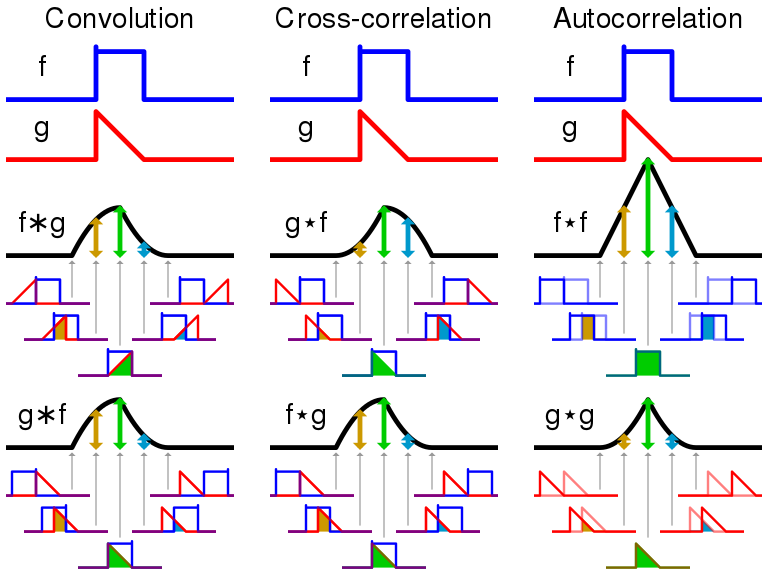自相关分析是一种数学工具，可用于查找重复模式，例如是否存在被噪声掩盖的周期性信号，或在其谐波频率所隐含的信号中识别缺少的基频。 它通常用于信号处理中，以分析功能或一系列值，例如时域信号。 不同的研究领域对自相关的定义不同，并且并非所有这些定义都是等效的。 在某些领域，该术语可与自协方差（autocovariance）互换使用。 单位根过程，趋势平稳过程，自回归过程和移动平均过程是具有自相关过程的特定形式。
一般定义
在统计中，实数或复数随机过程的自相关是两个不同时间序列或者时滞长度（两个时间序列的差）的函数的皮尔逊相关性。假设{Xt}是随机过程，而{t}是任何时间点（{t}可以是离散时间过程的整数，或者连续时间过程的实数）。然后{Xt}是在时间t时刻给定进程运行所产生的值。假设对于每个时刻t，过程都具有均值和方差。那么，时间t1和t2之间的自相关函数的定义为：
（1）
其中E是期望值运算符，*表示复数的共轭。请注意，期望可能没有很好地定义。在相乘前减去平均值会在时间t1和t2之间产生自协方差函数：
（2）
请注意，这个表达式并不适用于所有时间序列或过程，因为均值可能不存在，或者方差可能为零（对于恒定过程）或无限（对于分布缺少行为良好的矩的过程，例如某些类型的幂定律）。
广义平稳随机过程的定义
如果{Xt}是广义平稳过程，则均值和方差与时间无关，此外，自协方差函数（autocovariance）仅取决于t1和t2之间的时差：自协方差仅取决于时间距离在两个值之间，而不是绝对时间。这个结论的前提是随机过程是一个广义平稳随机过程。
这进一步暗示可以将自协方差和自相关表示为时滞的函数，并且这将是时滞的偶数函数。这给出了更为我们熟悉的自相关函数（auto-correlation function）的形式：
（3）
和自协方差函数（auto-covariance function）：
（4）
这个形式其实是统计物理中我们所用到的所谓自关联函数，或者自相关函数（这种称呼的前提一般是假定实过程均值为0），而实际的称呼应该为自协方差函数。
归一化形式
在不同学科的实际应用中，经常会通过归一化自协方差函数从而得到与时间有关的皮尔逊相关系数（Pearson correlation coefficient）。然而，在其他学科（例如工程学）中，通常不使用归一化，并且术语“自相关”和“自协方差”可互换使用。
随机过程的自相关系数（auto-correlation coefficient）的定义为：
（5）
如果函数定义明确，则其值必须在[ -1,1]之中，其中1表示完全相关，而-1表示完全负相关。
对于弱平稳过程（weak-sense stationarity process）和广义平稳过程（wide-sense stationarity process），定义为：
（6）
其中：

归一化很重要，因为自相关的解释为相关性提供了对统计依赖强度的无标度度量，并且归一化会对所估计的自相关统计特性产生影响。
自相关函数属性
对称性
自相关函数Rxx是一个偶函数：

在弱平稳过程中（WSS）：

零点取最大值
对于WSS过程：
注意恒为实数。

柯西-施瓦茨不等式（Cauchy-Schwarz inequality）

维纳-辛钦定理（Wiener-Khinchin Theorem）

对于一个实过程，其自相关函数有一个实对称的变换，上述公式可以简化为：

确定信号下的自相关函数
在信号处理中，上面的定义经常不经归一化使用，即不减去均值并除以方差。当通过均值和方差对自相关函数进行归一化时，有时将其称为自相关系数或自协方差函数。
连续时间信号的自相关
给定信号f(t)的情况下，连续自相关函数通常定义为在时滞下对f(t)的互关联函数（cross-correlation）的连续积分：

其中f*(t)表示f(t)的共轭。请注意，积分中的参数t是一个虚拟变量，仅在计算积分时才需要。它没有特定的含义。
离散时间信号的自相关
给定离散时间信号y(n)，时滞l下的离散自相关R为：

上面的定义适用于平方可积或者具有有限平方和的信号，即具有有限的能量。相反，“永远持续”的信号被视为随机过程，在这种情况下，需要基于期望值给出不同的定义。对于广义平稳随机过程，自相关定义为：

对于一个非平稳的过程，上述为t或n的函数。
如果这个过程具有遍历性，则其期望E可以被时间上的平均所代替。对于一个具有遍历性的过程，其自相关还可以定义为：

这些定义的优点是，即使周期函数不是平稳的遍历过程的输出，它们也可以为周期函数提供合理的，定义明确的单参数结果。
注意上述内容即是统计物理里面的自相关定义，及由于遍历性导致的自相关在某个点上的平均等价于在时间序列上的平均。这种等价的前提是信号本身具有平方不可积\求和的特点。
显然根据上述的定义orign软件中使用的公式是错误的，即其没有对总时间T或总个数N求和。这是非常注意的一点，当然这种错误只差一个系数，而不对定性产生影响，但会对量级产生影响。
另外，可以使用有限时间积分通过短时自相关函数分析来处理无穷长信号。
周期信号的定义
如果f是一个周期为T的连续周期函数，则其内的积分可以被替换为的积分：

上式等价于：

其中意为“记作”、“定义为”、“等价于”。
属性
下面，我们将仅描述一维自相关的属性，因为大多数属性很容易从一维情况转移到多维情况。这些特性适用于广义的平稳过程。
1）自相关的基本属性是对称，，这很容易从定义中得到证明。在连续情况下，当f是实函数时,自相关为偶函数:

当f为复数函数时，自相关函数为厄米函数（Hermitian function）：

2）连续自相关函数在原点达到峰值，在此取绝对值，即对于任何有：

这是重排不等式的结果。在离散情况下，结果相同。
3）周期函数的自相关本身就是具有相同周期的周期。
4）两个完全不相关的函数之和的自相关（对所有的互相关为零）是每个函数的自相关的和。
5）由于自相关是互相关（cross-correlation）的一种特定类型，因此它保留了互相关的所有属性。
6）通过使用符号*来表示卷积，而是操纵函数f的函数，并定义为:

则可以表示为：


展开全文数据分析
• 随机信号也称为随机过程、随机函数或随机序列。 确定性信号:如果序列{s(t)}在每个时刻的取值不是随机的，而是服从某种固定函数的关系，则称之为确定性信号。如阶跃信号、符号信号或矩形脉冲等。 随机信号：若序列{...
一、涉及的概念
随机：随机是指信号的取值服从某种概率规律，这一规律可以是完全已知的、部分已知的或完全未知的。随机信号也称为随机过程、随机函数或随机序列。
确定性信号:如果序列{s(t)}在每个时刻的取值不是随机的，而是服从某种固定函数的关系，则称之为确定性信号。如阶跃信号、符号信号或矩形脉冲等。
随机信号：若序列{s(t)}在每个时刻的取值是随机变量，则称之为随机信号。随机信号过过程可以用概率分布特性（简称统计性能）统计地描述。
令x(t)表示一连续时间的复随机过程，对于任何一固定的时刻t，随机过程x(t）定义一随机变量X=x(t).令u(t)表示其均值，则：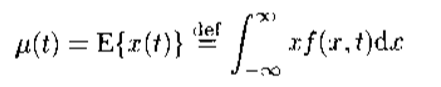式中f(x,t)表示随机变量X = x(t) 在时间t的概率密度函数。
自相关函数Rx(t1,t2)定义为x(t)在时刻t1和t2之间的相关，即：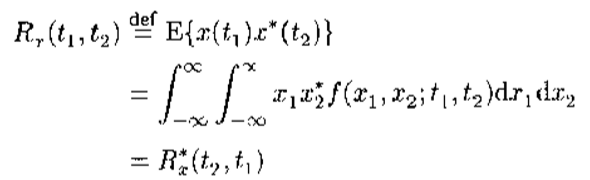式中，上标*表示复数的共轭；f(x1,x2;t1,t2)表示随机变量X1 = x(t1)和X2 = x(t2)的联合概率密度函数。一般情况下，自相关函数与时间变量t1和t2有关。
均值和自相关函数分别是随机信号x(t)的一阶矩和二阶矩，类似地还可以定义随机信号的K阶矩：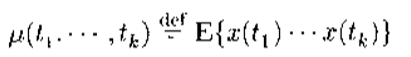根据K阶矩是否与时间有关，随机信号又分为平稳随机信号和非平稳随机信号两大类。
平稳信号:n阶平稳、广义平稳、严格平稳。平稳信号常称为时不变信号，即统计量不随时间变化的信号。
非平稳信号：也常称为时变信号，因为它至少有某个统计量（如均值，协方差函数）是时间的函数。
注意：时变与时不变信号不应该理解为信号的取值或波形是否随时间变化。
遍历性：它关心的问题是从随机信号的依次记录是否可以估计其统计量（如相关函数，功率谱等）。常用一种遍历性形式，均方遍历性。
当一个信号是n阶矩均方遍历的平稳过程时，它的n阶及所有低阶的统计平均都可以用各自的时间平均来代替。换句话说，这些统计量均可以根据该信号的一次观测数据进行估计。如果均方遍历的平稳信号x(t)的N个观测样本x(1),x(2),...,x(N),为已知，则信号的均值ux可由时间平均估计。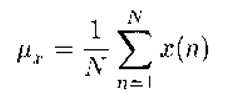统计特性：一阶、二阶、高阶（三阶及以上）

二、相关函数、协方差函数与功率谱密度
相关函数、协方差函数与功率谱密度是描述平稳随机信号统计特性最常用的二阶统计量。
2.1 单个随机信号x(t)——自相关函数、自协方差函数与功率谱密度

自相关函数、自协方差函数
令x(t)是一取复值的广义平稳随机信号，时间变量t属于负无穷—正无穷或者零—正无穷。意味着随机信号x(t)的均值与时间t无关，为常数。令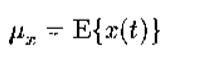则x(t)在t1和t2的自相关函数和自协方差函数仅决定于时间差t1-t2,分别定义为;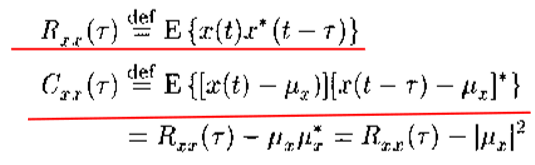自相关函数和自协方差函数常称为相关函数和协方差函数，它们的变元τ表示两个信号取值的时间差，称为滞后。
（1）对于具有零均值的随机信号x(t)而言，自协方差函数与自相关函数等价；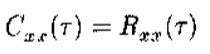（2）当τ=0时，信号x(t)的自相关函数退化为x(t)的二阶矩，即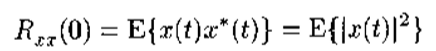（3）当τ = 0时，信号x(t)的自协方差函数退化为x(t)的方差，即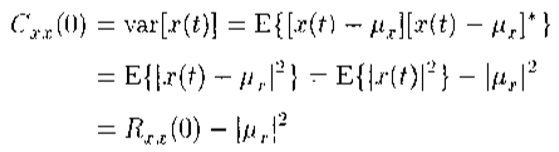功率谱密度: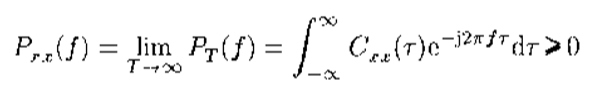性质：
（1）功率谱密度是实的；
（2）功率谱密度是非负的，即大于等于零；
（3）自协方差函数是功率谱密度的傅里叶反变换，即;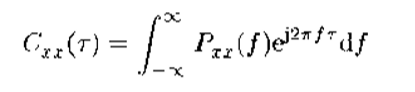（4）若x(t)是零均值的随机过程，则协方差函数与相关函数等价，此时;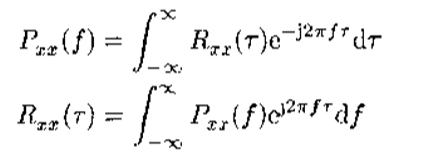实际上，这个关系就是维纳辛钦定理。
文字表述为：任意一个零均值的广义平稳随机过程的功率谱和它的自相关函数组成一个傅里叶变换对。
若功率谱等于常数，则随机过程称为白噪声；之所以称为白噪声是因为它的功率（或能量）与频率无关，具有与白色光相同的能量分布性质。相反，功率谱不等于常数的噪声称为有色噪声。

自相关函数矩阵：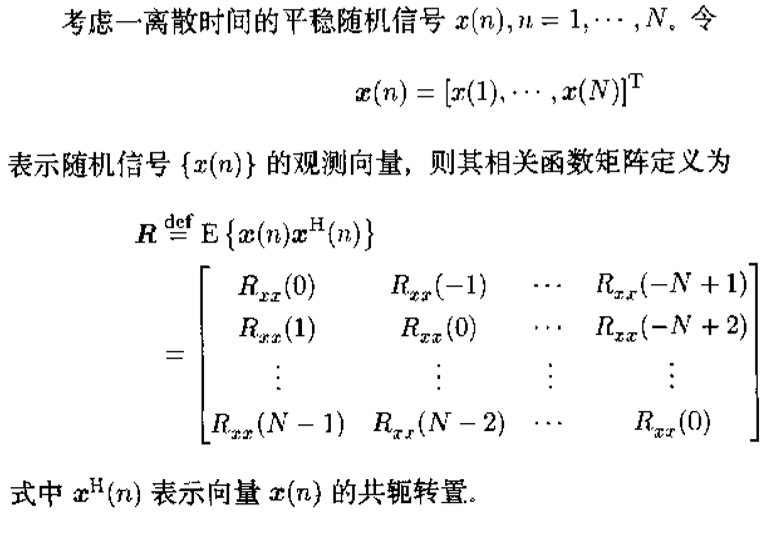这种矩阵叫做Toeplitz矩阵，对角线元素相同，而且其他对角线也分别具有相同的元素。 由于Rxx（-K）= Rxx*（K），故一个N*N阶的Toeplitz矩阵由它的N个自相关函数Rxx（0）,Rxx（1）,...Rxx（N-1）所决定。
半正定矩阵：所有特征值是正的或零值，不能为负值。半正定性是相关函数矩阵具有的一个重要性质。

2.2 两个随机信号x(t)和y(t)——互相关函数、互协方差函数与功率谱密度
互相关函数、互协方差函数：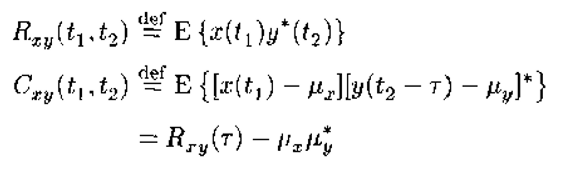互相关系数：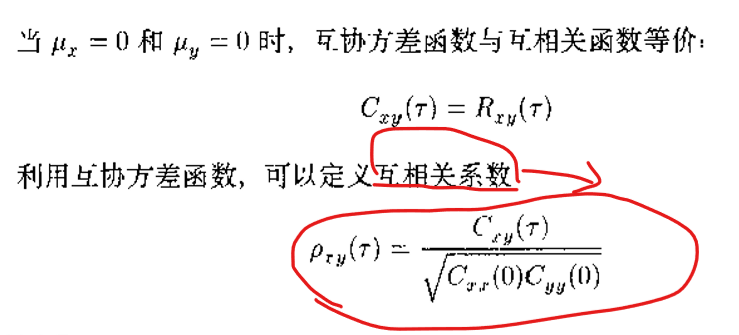互相关系数物理解释：
互协方差函数涉及两个不同信号x(t)和y(t)之间的相乘，一般来说，这两个减去均值的信号存在共性部分（确定量）和非共性部分（随机量），而共性部分的相乘总是去相同的符号，使得该部分得到加强而保留下来。与之不同，两个信号的非共性部分是随机的，它们的乘积有时取负，有时取正，通过数学期望的平均运算后，趋于相互“抵消”。
这意味着，互协方差函数能够把两个信号之间共性部分提取出来，并抑制掉非共性部分。因此，互协方差函数描述了两个信号之间的相关（联）程度。但是这种相关程度是用绝对量来衡量的，并不方便。对互协方差函数做归一化处理，得到互相关系数后，两个信号之间的相关程度就很容易度量了。
若互相关系数越接近于1，则两个信号越相似；反之，互相关系数越接近于0，两个信号的差异就越大。

互功率谱密度：定义为互协方差函数的傅里叶变换；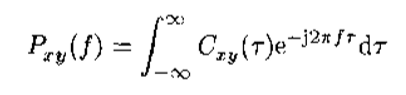互功率谱密度的实部称为同相谱，虚部称为正交谱，记为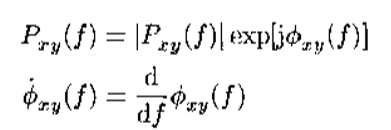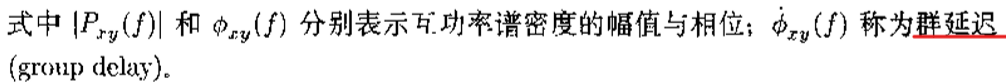相干函数：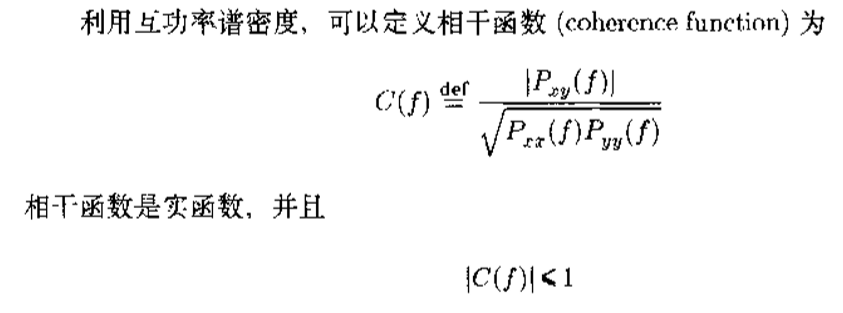2.3 小结
相关函数、协方差函数及相关系数等描述信号在时间域（主要是滞后）的统计特性，属于时域特性；而功率谱密度和相干函数等则描述信号在频率域的统计性质，归为频域特性。由于协方差函数和功率谱密度可以通过傅里叶变换相互转换，所以信号的时域特性和频域特性具有同等重要的作用。
概率密度分布服从正态分布的随机信号称为高斯随机信号，而具有非正态分布的随机信号称为非高斯随机信号。对于非高斯信号，仅使用相关函数和功率谱是不能完全描述信号的统计性质的，这是需要用到三阶甚至更高阶的统计量，它们统称为高阶统计量。包含高阶矩，高阶累积量，高阶谱等。
在进行平稳信号的处理之前，通常需要先估计信号的均值，然后对每个信号值减去该均值。这一处理称为平稳信号的零均值化。由于零均值化是信号处理的必然预处理，所以在很多文献中将相关函数和协方差函数混用，因为零均值信号的相关函数和协方差函数二者是等价的。
三、两个信号的比较与识别
3.1 独立、不相关、正交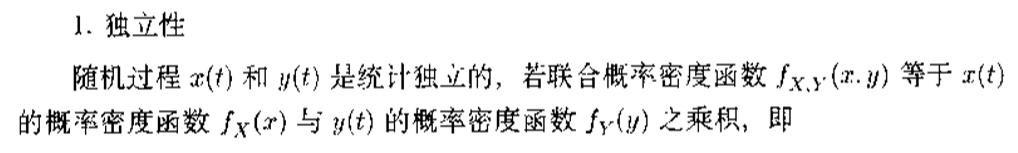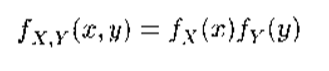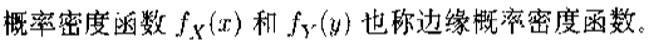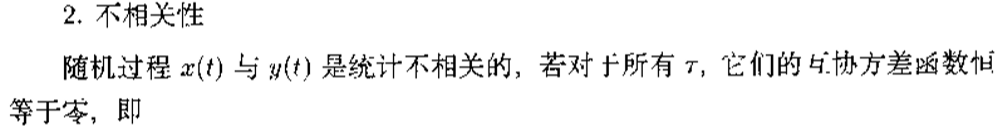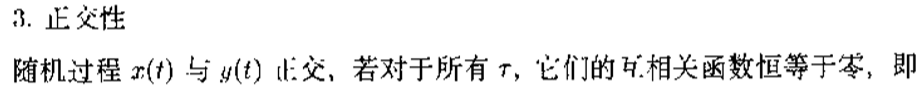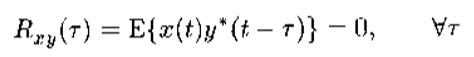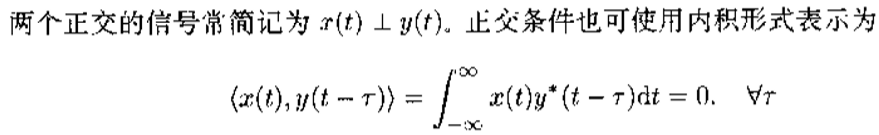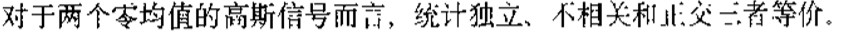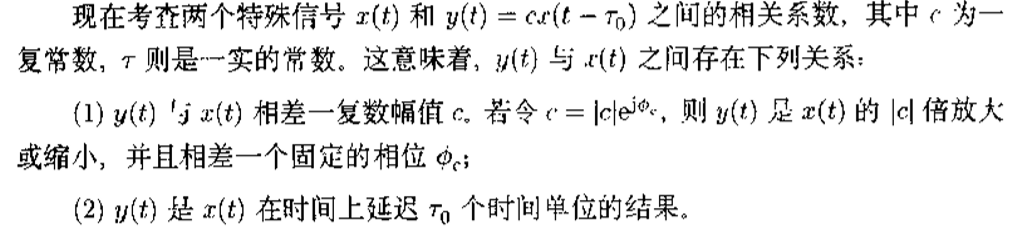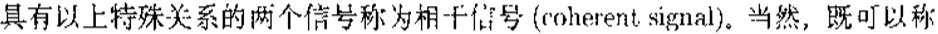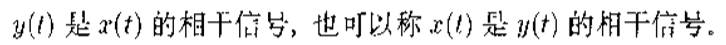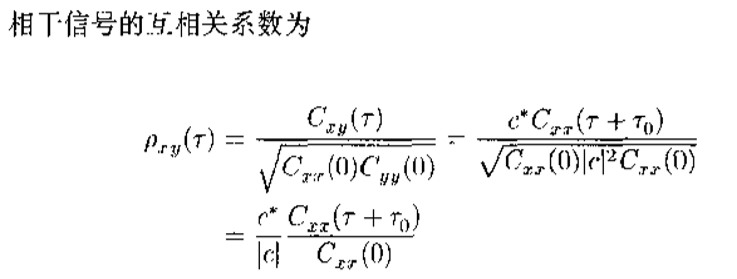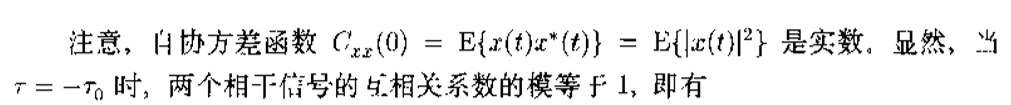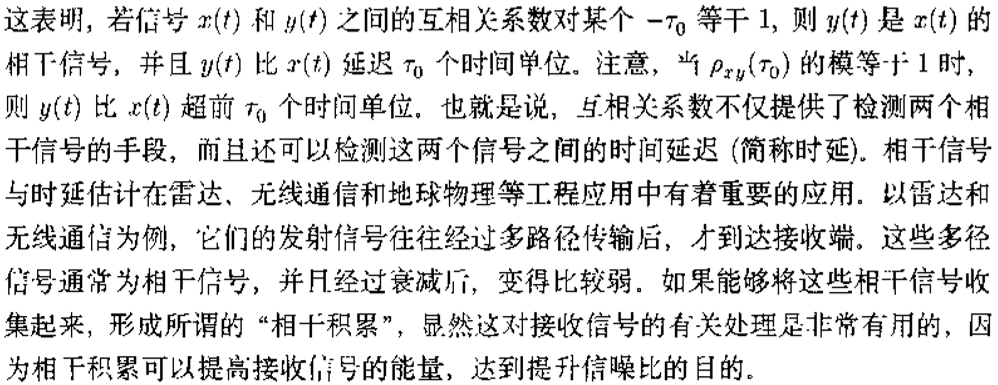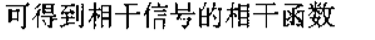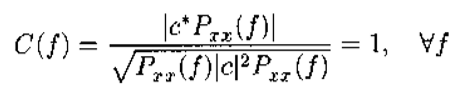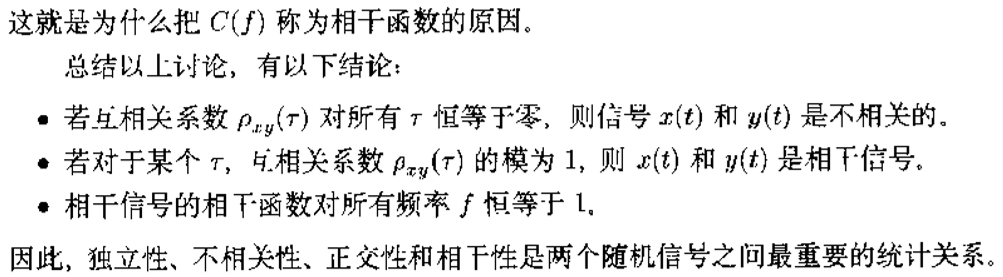3.2 信号变换
同时适用于均匀和非均匀采样信号的工具。
如：傅里叶变换、K-L变换
（1）将一个信号展开为级数形式, φk有何种要求？Ck如何确定？展开系数Ck是使用信号x(t）某种积分形式来确定的，这一积分分式习惯称为信号变换。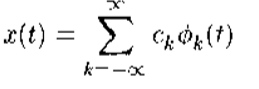（2）如傅里叶变换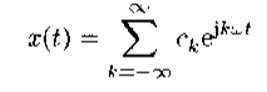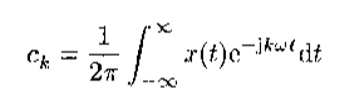这个就是信号变换公式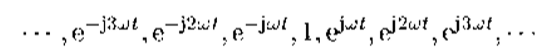称为傅里叶展开的基函数
（3）使用正交基函数的信号变换称为正交信号变换；使用非正交基函数的信号变换称为非正交信号变换；

3.3 多项式序列的斯密特标准正交化
四、具有随机输入的线性系统
（1）系统输出的功率谱密度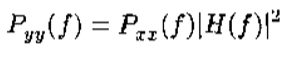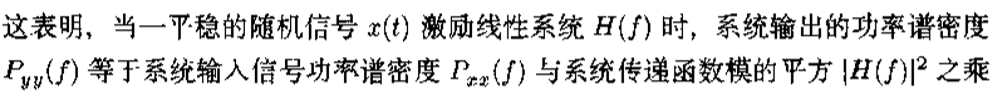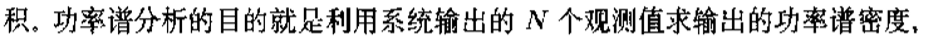（2）窄带功率谱估计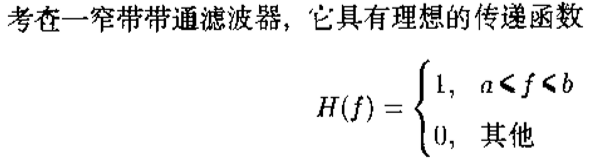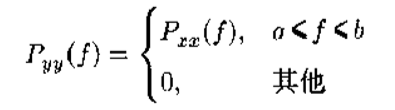申明:内容依据张贤达教授所著《现代信号处理》第二版所总结归纳
展开全文• 本文主要介绍了AR(p)序列的自协方差函数与谱密度函数，以及两者之间的关系。


Contents

A

R

(

p

)

{\rm AR}(p)

序列的自协方差函数与谱密度

A

R

(

p

)

{\rm AR}(p)

序列的自协方差函数Yule-Walker 方程

A

R

(

p

)

{\rm AR}(p)

序列的谱密度谱密度的自协方差函数反演公式

A

R

(

p

)

{\rm AR}(p)

序列的自协方差函数与谱密度

A

R

(

p

)

{\rm AR}(p)

序列的自协方差函数
写出

A

R

(

p

)

{\rm AR}(p)

模型的平稳解为

X

t

=

A

−

1

(

B

)

ϵ

t

=

∑

j

=

0

∞

ψ

j

ϵ

t

−

j

,

t

∈

Z

.

X_t=A^{-1}(\mathscr{B})\epsilon_t=\sum_{j=0}^\infty\psi_j\epsilon_{t-j} \ , \ \ \ \ t\in\Z.

由线性平稳序列的性质知

{

X

t

}

\{X_t\}

为零均值

E

(

X

t

)

=

0

{\rm E}(X_t)=0

，且有自协方差函数

γ

k

=

E

(

X

t

+

k

X

t

)

=

σ

2

∑

j

−

0

∞

ψ

j

ψ

j

+

k

,

k

=

0

,

1

,

2

,

.

.

.

\gamma_k={\rm E}(X_{t+k}X_t)=\sigma^2\sum_{j-0}^\infty\psi_j\psi_{j+k} \ , \ \ \ \ k=0,1,2,...

之前已经证明 Wold 系数的负指数阶收敛性

ψ

j

=

o

(

ρ

−

j

)

\psi_j=o(\rho^{-j})

，

1

<

ρ

<

min

⁡

{

∣

z

j

∣

}

1<\rho<\min\{|z_j|\}

，因此有

∣

γ

k

∣

≤

σ

2

∑

j

=

0

∞

∣

ψ

j

∣

∣

ψ

j

+

k

∣

≤

σ

2

(

∑

j

=

0

∞

ψ

j

2

∑

j

=

0

∞

ψ

j

+

k

2

)

1

2

≤

c

0

(

∑

j

=

k

∞

ρ

−

2

j

)

1

2

≤

c

1

ρ

−

k

|\gamma_k|\leq\sigma^2\sum_{j=0}^\infty|\psi_j||\psi_{j+k}|\leq\sigma^2\left(\sum_{j=0}^\infty\psi_j^2\sum_{j=0}^\infty\psi_{j+k}^2\right)^{\frac12}\leq c_0\left(\sum_{j=k}^\infty\rho^{-2j}\right)^\frac12\leq c_1\rho^{-k}

其中

c

0

,

c

1

c_0,\,c_1

为正常数。因此

A

R

(

p

)

{\rm AR}(p)

序列的自协方差函数

{

γ

k

}

\{\gamma_k\}

也是以负指数阶收敛到

0

0

的，且

min

⁡

{

∣

z

j

∣

}

\min\{|z_j|\}

越大，

ψ

k

\psi_k

的收敛速度越快。说明

A

R

(

p

)

{\rm AR}(p)

序列前后的相关性减少很快，称为时间序列的短记忆性。
Yule-Walker 方程
首先我们需要补充说明平稳解的合理性，即说明

t

t

时刻的随机现象

X

t

X_t

和将来的白噪声

ϵ

t

+

k

,

k

≥

1

\epsilon_{t+k},\ k\geq1

无关。我们对平稳解的两边同乘

ϵ

t

+

k

\epsilon_{t+k}

并取数学期望，由控制收敛定理得到

E

(

X

t

ϵ

t

+

k

)

=

∑

j

=

0

∞

ψ

j

E

(

ϵ

t

−

j

ϵ

t

+

k

)

=

0

,

k

≥

1.

{\rm E}(X_t\epsilon_{t+k})=\sum_{j=0}^\infty\psi_j{\rm E}(\epsilon_{t-j}\epsilon_{t+k})=0 \ , \ \ \ \ k\geq1.

特别地，如果

{

ϵ

t

}

\{\epsilon_t\}

是独立白噪声，则

X

t

X_t

和将来的白噪声独立。
本章中我们先给出 Yule-Walker 方程的表现形式，然后在给予推导。

形式一：

A

R

(

p

)

{\rm AR}(p)

序列的自协方差函数满足

A

R

(

p

)

{\rm AR}(p)

模型

A

(

B

)

X

t

=

ϵ

t

A(\mathscr{B})X_t=\epsilon_t

相应的其次差分方程

γ

k

=

a

1

γ

k

−

1

+

a

2

γ

k

−

2

+

.

.

.

+

a

p

γ

k

−

p

,

k

≥

1

\gamma_k=a_1\gamma_{k-1}+a_2\gamma_{k-2}+...+a_p\gamma_{k-p} \ , \ \ \ \ k\geq1

γ

0

=

a

1

γ

1

+

a

2

γ

2

+

.

.

.

+

a

p

γ

p

+

σ

2

\gamma_0=a_1\gamma_1+a_2\gamma_2+...+a_p\gamma_p+\sigma^2

形式二：

A

R

(

p

)

{\rm AR}(p)

序列的自协方差函数满足如下方程

γ

n

=

Γ

n

a

n

,

n

≥

p

\boldsymbol{\gamma}_n=\boldsymbol\Gamma_n\boldsymbol{a}_n\ , \ \ \ \ n\geq p

γ

0

=

γ

n

T

a

n

+

σ

2

,

n

≥

p

\gamma_0=\boldsymbol\gamma_n^{\rm T}\boldsymbol{a}_n+\sigma^2 \ , \ \ \ \ n\geq p

其中，自协方差矩阵和自协方差向量定义如下：

Γ

n

=

[

γ

0

γ

1

⋯

γ

n

−

1

γ

1

γ

0

⋯

γ

n

−

2

⋮

⋮

⋮

γ

n

−

1

γ

n

−

2

⋯

γ

0

]

γ

n

=

[

γ

1

γ

2

⋮

γ

n

]

\boldsymbol\Gamma_n=\left[ \begin{array}{cccc} \gamma_0 & \gamma_1 & \cdots & \gamma_{n-1} \\ \gamma_1 & \gamma_0 & \cdots & \gamma_{n-2} \\ \vdots & \vdots & & \vdots \\ \gamma_{n-1} & \gamma_{n-2} & \cdots & \gamma_0 \\ \end{array} \right] \ \ \ \ \ \ \ \ \boldsymbol\gamma_n=\left[ \begin{array}{cccc} \gamma_1 \\ \gamma_2 \\ \vdots \\ \gamma_n \\ \end{array} \right]

自回归系数向量定义如下：

a

n

=

(

a

n

,

1

,

a

n

,

2

,

.

.

.

,

a

n

,

n

)

T

≜

(

a

1

,

a

2

,

.

.

.

,

a

p

,

0

,

0

,

.

.

.

,

0

)

T

,

n

≥

p

\boldsymbol{a}_n=(a_{n,1},a_{n,2},...,a_{n,n})^{\rm T} \triangleq(a_1,a_2,...,a_p,0,0,...,0)^{\rm T} \ , \ \ \ \ n\geq p

这一形式和偏相关系数的定义有关，体现了偏相关系数

p

p

步截尾的性质，会在后续内容中介绍。

形式三：对

k

<

0

k<0

，定义

ψ

k

=

0

\psi_k=0

，可以把 Yale-Walker 方程写成更一般的形式

γ

k

−

(

a

1

γ

k

−

1

+

a

2

γ

k

−

2

+

.

.

.

+

a

p

γ

k

−

p

)

=

σ

2

ψ

−

k

,

k

∈

Z

\gamma_k-(a_1\gamma_{k-1}+a_2\gamma_{k-2}+...+a_p\gamma_{k-p})=\sigma^2\psi_{-k} \ , \ \ \ \ k\in\Z

形式一的证明：
写出

A

R

(

p

)

{\rm AR}(p)

模型的定义：

X

t

=

∑

j

=

1

p

a

j

X

t

−

j

+

ϵ

t

,

t

∈

Z

.

X_t=\sum_{j=1}^pa_jX_{t-j}+\epsilon_t\ , \ \ \ \ t\in\Z.

对模型的两边同乘

X

t

−

k

X_{t-k}

并取数学期望

E

(

X

t

X

t

−

k

)

=

∑

j

=

1

p

a

j

E

(

X

t

−

j

X

t

−

k

)

+

E

(

ϵ

t

X

t

−

k

)

{\rm E}(X_tX_{t-k})=\sum_{j=1}^pa_j{\rm E}(X_{t-j}X_{t-k})+{\rm E}(\epsilon_tX_{t-k})

结合将来的白噪声不能决定过去的随机现象，即

E

(

ϵ

t

X

t

−

k

)

=

0

{\rm E}(\epsilon_tX_{t-k})=0

，上式可以化简为

γ

k

=

a

1

γ

k

−

1

+

a

2

γ

k

−

2

+

.

.

.

+

a

p

γ

k

−

p

,

k

≥

1

\gamma_k=a_1\gamma_{k-1}+a_2\gamma_{k-2}+...+a_p\gamma_{k-p} \ , \ \ \ \ k\geq1

接下来求

γ

0

\gamma_0

满足的式子：
对模型的两边同乘

X

t

X_{t}

并取数学期望

E

(

X

t

2

)

=

∑

j

=

1

p

a

j

E

(

X

t

X

t

−

j

)

+

E

(

ϵ

t

X

t

)

{\rm E}(X_t^2)=\sum_{j=1}^pa_j{\rm E}(X_tX_{t-j})+{\rm E}(\epsilon_tX_t)

γ

0

=

∑

j

=

1

p

a

j

γ

j

+

E

[

ϵ

t

⋅

(

∑

j

=

1

p

a

j

X

t

−

j

+

ϵ

t

)

]

=

∑

j

=

1

p

a

j

γ

j

+

∑

j

=

1

p

a

j

E

(

ϵ

t

X

t

−

j

)

+

E

ϵ

t

2

=

∑

j

=

1

p

a

j

γ

j

+

0

+

σ

2

=

a

1

γ

1

+

a

2

γ

2

+

.

.

.

+

a

p

γ

p

+

σ

2

.

\begin{aligned} \gamma_0&=\sum_{j=1}^pa_j\gamma_j+{\rm E}\left[\epsilon_t\cdot\left(\sum_{j=1}^pa_jX_{t-j}+\epsilon_t\right)\right] \\ &=\sum_{j=1}^pa_j\gamma_j+\sum_{j=1}^pa_j\,{\rm E}\left(\epsilon_tX_{t-j}\right)+{\rm E}\epsilon_t ^2 \\ &=\sum_{j=1}^pa_j\gamma_j+0+\sigma^2 \\ &=a_1\gamma_1+a_2\gamma_2+...+a_p\gamma_p+\sigma^2. \end{aligned}

形式二的证明：
对

n

≥

p

n\geq p

，将

A

R

(

p

)

{\rm AR}(p)

模型的定义式写成矩阵形式：

[

X

t

X

t

+

1

⋮

X

t

+

n

−

1

]

=

[

X

t

−

1

X

t

−

2

⋯

X

t

−

n

X

t

X

t

−

1

⋯

X

t

+

1

−

n

⋮

⋮

⋮

X

t

+

n

−

2

X

t

+

n

−

3

⋯

X

t

−

1

]

a

n

+

[

ϵ

t

ϵ

t

+

1

⋮

ϵ

t

+

n

−

1

]

\left[ \begin{array}{c} X_t \\ X_{t+1} \\ \vdots \\ X_{t+n-1} \\ \end{array} \right]=\left[ \begin{array}{c} X_{t-1} & X_{t-2} & \cdots & X_{t-n} \\ X_t & X_{t-1} & \cdots & X_{t+1-n} \\ \vdots & \vdots & &\vdots \\ X_{t+n-2} & X_{t+n-3} & \cdots & X_{t-1} \\ \end{array} \right]\boldsymbol{a}_n+\left[ \begin{array}{c} \epsilon_t \\ \epsilon_{t+1} \\ \vdots \\ \epsilon_{t+n-1} \\ \end{array} \right]

对上式的两边同乘

X

t

−

1

X_{t-1}

并取数学期望即可得到 Y-W 方程：

γ

n

=

Γ

n

a

n

,

n

≥

p

\boldsymbol{\gamma}_n=\boldsymbol\Gamma_n\boldsymbol{a}_n\ , \ \ \ \ n\geq p

同理我们还需要求

γ

0

\gamma_0

满足的方程，对模型的两边同时平方并取数学期望：

γ

0

=

E

(

X

t

2

)

=

E

(

∑

j

=

1

p

a

j

X

t

−

j

+

ϵ

t

)

2

=

E

(

∑

j

=

1

p

a

j

X

t

−

j

)

2

+

E

ϵ

t

2

=

E

(

∑

j

=

1

n

a

n

,

j

X

t

−

j

)

2

+

E

ϵ

t

2

=

a

n

T

Γ

n

a

n

+

σ

2

=

a

n

T

γ

n

+

σ

2

,

n

≥

p

.

\begin{aligned} \gamma_0={\rm E}(X_t^2) &={\rm E}\left(\sum_{j=1}^pa_jX_{t-j}+\epsilon_t\right)^2 \\ &={\rm E}\left(\sum_{j=1}^pa_jX_{t-j}\right)^2+{\rm E}\epsilon_t^2 \\ &={\rm E}\left(\sum_{j=1}^na_{n,j}X_{t-j}\right)^2+{\rm E}\epsilon_t^2 \\ &=\boldsymbol{a}_n^{\rm T}\boldsymbol{\Gamma}_n\boldsymbol{a}_n+\sigma^2 \\ \\ &=\boldsymbol{a}_n^{\rm T}\boldsymbol{\gamma}_n+\sigma^2 \ , \ \ \ \ n\geq p . \end{aligned}

形式三的证明：
对

k

≥

0

k\geq0

，前两个形式的 Y-W 方程已经得证，下面推导

k

<

0

k<0

的部分。
依然对

A

R

(

p

)

{\rm AR}(p)

模型的两边同乘

X

t

−

k

X_{t-k}

取数学期望，但需要稍微移项：

γ

k

−

(

a

1

γ

k

−

1

+

a

2

γ

k

−

2

+

.

.

.

+

a

p

γ

k

−

p

)

=

E

[

X

t

−

k

(

X

t

−

∑

j

=

1

p

a

j

X

t

−

j

)

]

=

E

[

X

t

−

k

ϵ

t

]

=

E

[

ϵ

t

⋅

∑

j

=

0

∞

ψ

j

ϵ

t

−

k

−

j

]

=

σ

2

ψ

−

k

\begin{aligned} \gamma_k-(a_1\gamma_{k-1}+a_2\gamma_{k-2}+...+a_p\gamma_{k-p}) &={\rm E}\left[X_{t-k}(X_t-\sum_{j=1}^pa_jX_{t-j})\right]\\ \\ &={\rm E}\left[X_{t-k}\epsilon_t\right]\\ \\ &={\rm E}\left[\epsilon_{t}\cdot\sum_{j=0}^\infty\psi_j\epsilon_{t-k-j}\right] \\ \\ &=\sigma^2\psi_{-k} \end{aligned}

最后需要将

A

R

(

p

)

{\rm AR}(p)

模型的平稳解代入，即可得证。
Y-W 方程还可以用推移算子多项式写为：

A

(

B

)

γ

k

=

σ

2

ψ

−

k

,

k

∈

Z

A(\mathscr{B})\gamma_k=\sigma^2\psi_{-k}\ , \ \ k\in\Z

。

A

R

(

p

)

{\rm AR}(p)

序列的谱密度
利用线性平稳序列的谱密度公式可以得到

A

R

(

p

)

{\rm AR}(p)

序列的谱密度

f

(

λ

)

=

σ

2

2

π

∣

∑

j

=

0

∞

ψ

j

e

i

j

λ

∣

2

=

σ

2

2

π

∣

A

(

e

i

λ

)

∣

2

.

f(\lambda)=\frac{\sigma^2}{2\pi}\left|\sum_{j=0}^\infty\psi_je^{ij\lambda}\right|^2=\frac{\sigma^2}{2\pi\left|A(e^{i\lambda})\right|^2}.

f

(

λ

)

f(\lambda)

是一个恒正的偶函数。通过谱密度的表达式可以看出，如果特征多项式

A

(

z

)

A(z)

有复根

z

j

=

ρ

j

e

i

λ

j

z_j=\rho_je^{i\lambda_j}

靠近单位圆，则谱密度

f

(

λ

)

f(\lambda)

会在

λ

j

\lambda_j

附近表现出峰值。
谱密度的自协方差函数反演公式
根据 Herglotz 定理推论，平稳序列的谱密度如果存在则在几乎处处的意义下是唯一的。回顾谱密度的定义式，

γ

k

=

∫

−

π

π

f

(

λ

)

e

i

k

λ

d

λ

,

k

∈

Z

.

\gamma_k=\int_{-\pi}^\pi f(\lambda)e^{ik\lambda}\,{\rm d}\lambda \ , \ \ \ \ k\in\Z.

根据这一定义，我们可以理解为利用平稳序列唯一存在的谱密度，我们可以计算出任意阶的自协方差函数。那么已知平稳序列任意阶的自协方差函数，是否可以计算该平稳序列的谱密度呢？事实上，在自协方差函数绝对可和的条件下，平稳序列的谱密度可以由它的自协方差函数表示出来。我们引入谱密度的自协方差函数反演公式。

如果平稳序列

{

X

t

}

\{X_t\}

的自协方差函数

{

γ

k

}

\{\gamma_k\}

绝对可和，即

∑

k

=

−

∞

∞

∣

γ

k

∣

<

∞

\displaystyle\sum_{k=-\infty}^\infty|\gamma_k|<\infty

，则

{

X

t

}

\{X_t\}

有谱密度

f

(

λ

)

=

1

2

π

∑

k

=

−

∞

∞

γ

k

e

−

i

k

λ

.

f(\lambda)=\frac1{2\pi}\sum_{k=-\infty}^\infty\gamma_ke^{-ik\lambda}.

由于谱密度函数是实值函数，因此上式可以改写为

f

(

λ

)

=

1

2

π

∑

k

=

−

∞

∞

γ

k

cos

⁡

(

k

λ

)

=

1

2

π

[

γ

0

+

2

∑

k

=

1

∞

γ

k

cos

⁡

(

k

λ

)

]

f(\lambda)=\frac1{2\pi}\sum_{k=-\infty}^\infty\gamma_k\cos(k\lambda)=\frac1{2\pi}\left[\gamma_0+2\sum_{k=1}^\infty\gamma_k\cos(k\lambda)\right]

利用反演公式，

A

R

(

p

)

{\rm AR}(p)

序列的谱密度还可以写为

f

(

λ

)

=

σ

2

2

π

∣

∑

j

=

0

∞

ψ

j

e

i

j

λ

∣

2

=

σ

2

2

π

∣

A

(

e

i

λ

)

∣

2

=

1

2

π

∑

k

=

−

∞

∞

γ

k

e

−

i

k

λ

.

f(\lambda)=\frac{\sigma^2}{2\pi}\left|\sum_{j=0}^\infty\psi_je^{ij\lambda}\right|^2=\frac{\sigma^2}{2\pi\left|A(e^{i\lambda})\right|^2}=\frac1{2\pi}\sum_{k=-\infty}^\infty\gamma_ke^{-ik\lambda}.


展开全文• 协方差函数 在平稳 AR(p)AR(p)AR(p)模型两边同乘 xt−k,∀k&amp;amp;gt;1x_{t-k}, \forall k &amp;amp;gt; 1xt−k​,∀k&amp;gt;1， 再求期望 对于中心化的AR模型， 其均值为0, 则有cov(xt,xs)=E(xt)E...
• 由于时间序列通常具有较强的自相关性，我们通常使用自协方差函数与自相关函数（ACF）来描述时间序列。当时间序列具有宽平稳的性质时，可以使用样本数据来估计它们，下面介绍ACF(k)的计算方法。 假设时序   共有...时间序列 平稳性 python
• 二、自协方差函数（时间序列） (1)目标 a,记录各类随机变量，并且找出两个随机变量之间的协方差 b, 明确，时间序列描述为一系列随机过程的实现 c, 定义自协方差函数 (2)什么是协方差 X,Y 是两个随机变量，协方差则是...
• 在利用最小二乘配置进行GPS高程拟合时，协方差函数的确定直接关系到拟合的精度。提出了在预先不知道协方差函数具体形式的情况下，利用遗传规划的原理进行协方差函数的拟合。通过实例验证了遗传规划在协方差函数拟合...
• 协方差：引入协方差的公式 说明：这里有n个样本，计算时以n-1代替n是以较小的样本集逼近总体的标准差，即统计上的“无偏估计”，matlab中方差、标准差、协方差计算都是默认n-1，后面会有验证。 仿照方差的定义...matlab
• 1．自相关函数(Autocorrelation function)
• 顾及协方差函数影响的GPS高程拟合方法研究.pdf
• 一个 JAGS 模块，包含多个用于点参考数据分析的协方差函数，以及一个为区域数据分析的适当 CAR 模型创建精度矩阵的函数。开源软件
• 9.2.3自相关函数和自协方差函数  上面介绍的均值、均方值和方差描述的是一维随机变量的统计特性，不能反映不同时刻各数值之间的相互关系。例如，随机信号X(t)分别在t1，t2时刻的随机取值X(t1)，X(t2)之间的关联...
• 鉴于防止上篇文章太长，于是单独放一篇时间序列相关的重点概念理解。 另一部分内容????《【时序】思维导图、逻辑、解题步骤》 目录 1.时间序列分析 2. 平稳 （1）平稳性是什么含义？...3. 自协方差函数 1...
• 一：matlab里面关于方差和协方差的几点需要...求协方差函数：cov 1 如果a,b是向量，则： cov(a,b)相当于是[D(a),cov(a,b);cov(b,a),D(b)]，其中D(a)和D(b)分别代表了a,b的方差。cov(a,b)和cov(b,a)MATLAB
• ASWERS12345使用Somigliana公式计算GRS80自由空气异常，Eigen-CG03C和剩余地形减少模型，经验和解析协方差函数，正常重力（GRS67，GRS80）。 另一个 fftstokes.m 是使用 Stokes 公式的平面 FFT 计算大地水准面。 还...matlab
• z=[1,5;3,4;4,6;5,3]; ...%matlab函数结果 % b=cov(z) %  % 2.9167 -0.8333 % -0.8333 1.6667 %% z_mean=mean(z); result = zeros(2,2); for i = 1:2  for j=1:2  result(i,j)=(z(:,i
• 这个世界上最聪明的人就是会敲代码的，天呐，我死磕只能把自己搞坏了，有没有聪明的帮帮忙r语言
• 1　之前说过，运用统计分析常用的观测方式（观测尺度、观测量度）有均值、方差、协方差、自相关、偏相关。但是对于像时间序列这样一维的数据构成特点。有自有的自协方差、自相关和自偏相关，方式和方法也是引用统计...
• 1. 方差、协方差函数表 2. 名词解释 2.1 方差、标准差 2.2 无偏样本方差、无偏样本标准差 2.3总体协方差、样本协方差 3. 函数功能演示 3.1 方差与无偏方差 3.2 总体协方差与样本协方差 前言 在入门篇（十...大数据 hive 数据分析
• 在进行模式识别样本特征提取的时候，我们经常会用到协方差矩阵，它可以反映不同维度间的相关性。 一般来说，N x M的一个样本矩阵 （其中N ... 但是 matlab 的 cov 函数，计算矩阵的协方差时，采取的算法不同，cov(...matlab
• 一、源码 function [ COVMAT ] = covarianceMatrix( ...% 这是一个计算协方差矩阵的函数 % inputData 输入数据 % 每一行为一个维度 % 每一列为一个样本 %获得输入数据维度 [m,n] = size(inputData); %创建协...MATLAB
• 自相关函数 互相关函数协方差矩阵 互协方差矩阵的区别联系 : https://mermaidjs.github.io/ : http://adrai.github.io/flowchart.js/
• Matlab中cov函数详细解读 １、向量的方差与协方差矩阵 cov(x) 求向量x的方差。 cov(x)为一个数值，数值大小计算公式为S(x)。 cov(x,y) 求向量x与y的协方差矩阵。 cov(x,y)为2*2矩阵， [S(x) C(x,y); ......